Courses

# Revisal Problems (Past 13 Years) JEE Main (Hydrocarbons)

## 26 Questions MCQ Test Chemistry for JEE Advanced | Revisal Problems (Past 13 Years) JEE Main (Hydrocarbons)

Description
This mock test of Revisal Problems (Past 13 Years) JEE Main (Hydrocarbons) for JEE helps you for every JEE entrance exam. This contains 26 Multiple Choice Questions for JEE Revisal Problems (Past 13 Years) JEE Main (Hydrocarbons) (mcq) to study with solutions a complete question bank. The solved questions answers in this Revisal Problems (Past 13 Years) JEE Main (Hydrocarbons) quiz give you a good mix of easy questions and tough questions. JEE students definitely take this Revisal Problems (Past 13 Years) JEE Main (Hydrocarbons) exercise for a better result in the exam. You can find other Revisal Problems (Past 13 Years) JEE Main (Hydrocarbons) extra questions, long questions & short questions for JEE on EduRev as well by searching above.
QUESTION: 1

Solution:
QUESTION: 2

Solution:
QUESTION: 3

### Consider the following sequence of reaction,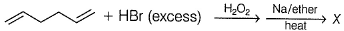Q. The product X is

Solution:
QUESTION: 4

A hydrocarbon on m onochlorin ation yields a single isom er while on dichlorination yielded three isomer (constitutional). The most likely hydrocarbon is

Solution:
QUESTION: 5

Which of the following on addition of bromine (Br2) will produce more than one isomers of dibromide?

Solution:
QUESTION: 6

Which of the following cyclic olefins would you predict to be aromatic?

Solution:
QUESTION: 7

Which of the following compounds will react most rapidly with acetyl chloride and aluminium chloride?

Solution:
QUESTION: 8

2 methylbutane on reacting with bromine in the presence of sunlight gives mainly

Solution:
QUESTION: 9

Which could be the major product of the following reaction?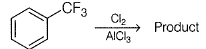Solution:
QUESTION: 10

Which of the following is not the resonance structure of intermediate from the listed electrophilic aromatic substitution?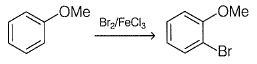Solution:
QUESTION: 11

What could be the product for the following reaction?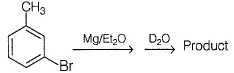Solution:
QUESTION: 12

Arrange the following alkene in increasing order of reactivity towards addition reaction with HCI.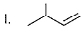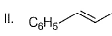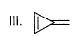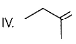Solution:
QUESTION: 13

In which of the following reaction, a visible change would-be observed?

Solution:
QUESTION: 14

If 2-methyl-2-butene is treated with NBS (N-bromo succinimide) in CCI4, how many mono-bromo alkenes would be produced in principle?

Solution:
QUESTION: 15

Which radical is responsible for the observed selectivity in the chain reaction below?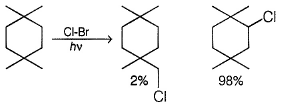Solution:
QUESTION: 16

What is the correct order of reactivity towards sulfonation reaction when treated with hot, conc, sulphuric acid solution?

Solution:
QUESTION: 17

Rank the following compounds in decreasing order of reactivity in electrophilic aromatic substitution reaction.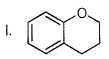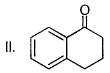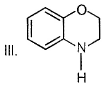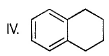Solution:
QUESTION: 18

Predict major product in the following reaction.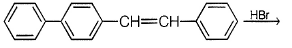Solution:
QUESTION: 19

Predict major product of the following reaction.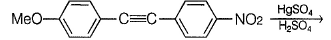Solution:
QUESTION: 20

What is the major product in the following reaction?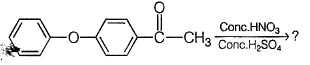Solution:
*Multiple options can be correct
QUESTION: 21

Direction (Q. Nos. 21-26) This section contains 6 multiple choice questions. Each question has four choices (a), (b), (c) and (dj, out of which ONE or MORE THAN ONE are correct.

Q. Which of the following can’t be produced as the single alkane in a Wurtz’s reaction?

Solution:
*Multiple options can be correct
QUESTION: 22

Which of the following will produce more than one alkane on reaction with ethanolic solution of KOH?

Solution:
*Multiple options can be correct
QUESTION: 23

If potassium propanoate is electrolysed in aqueous solution, the expected product(s) is/are

Solution:
*Multiple options can be correct
QUESTION: 24

Which of the following will produce more than one product on treatm ent with HgSO4/ H2SO4?

Solution:
*Multiple options can be correct
QUESTION: 25

Which of the following sets of reagent when applied sequentially on 2-butyne will produce a meso product?

Solution:
*Multiple options can be correct
QUESTION: 26

Select from the following molecules or ions which is/are aromatic?

Solution: